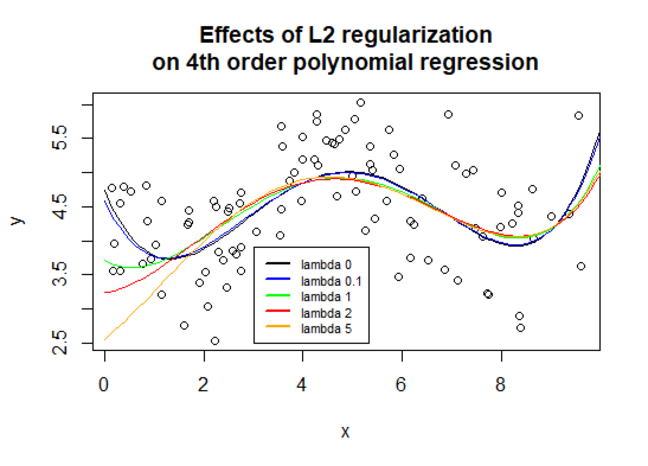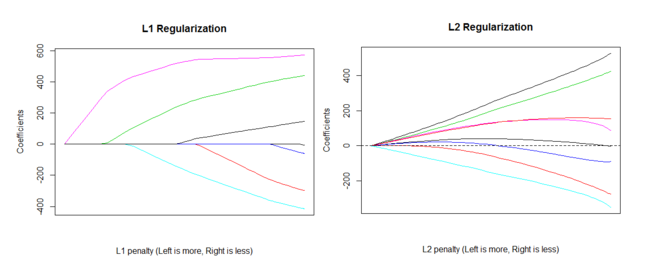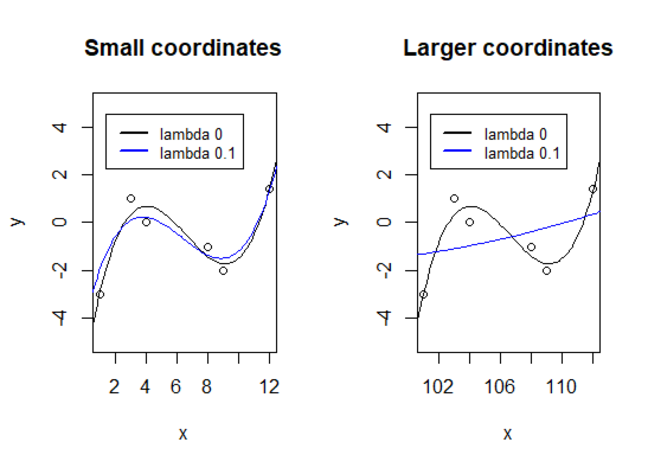## Want to keep learning?

This content is taken from the The Open University & Persontyle's online course, Advanced Machine Learning. Join the course to learn more.
1.19

# Regularization

If we define complexity as the number of parameters in a model, then managing complexity is difficult: It can only be achieved by effecting large scale changes to the model form. Consider the two simple modes we have used for most of our examples in this activity: Linear regression and logisitic regression (considered as a linear classifier):

Linear Regression: $$\hat y=\beta_0 x_0 + \beta_1 x_1 +\beta_2 x_2 + ... + \beta_n x_n$$

Logistic Regression: $$\hat y = \mathbf{I}(\beta_0 x_0 + \beta_1 x_1 +\beta_2 x_2 + ... + \beta_n x_n \geq 0)$$

Remembering that $$\mathbf{I}(p)=1$$ if $$p$$ is true and 0 otherwise.

In both these cases, if complexity is merely the number of parameters, altering complexity requires removing one of the input features. This is because each parameter is a coefficient of an input feature (treating the intercept, $$\beta_0$$ as a coefficient of the dummy variable $$x_0=1$$).

Equally, in more sophisticated models, removing parameters generally leads to some substantial alteration to the model form. In polynomial regression it requires removing one of the polynomial terms, In basic neural networks it will generally arise from removing hidden nodes, etc.

A crucial idea in advanced machine learning is that there is another, more nuanced, way of controlling the complexity of the model, still thinking of this as defined in terms of the parameters of a model. Rather than removing parameters, we can limit their ability to freely take on values. This is known as regularization.

## Regularization as optimization

In regularization theory, this is undertaken by adding a second term to the optimization problem being solved when fitting parameter values to data. We remember that the optimization problem was given in terms of minimizing some loss function:

$\hat\beta = argmin_\beta L(\beta,X,Y)$

Regularization theory adds a second term to this optimization problem, which we will term R:

$\hat\beta = argmin_\beta L(\beta,X,Y) + \lambda R(\beta)$

Note that $$R$$ is a function only of the parameters of the model. Two common regularization functions are L1 and L2 regularization:

$R_{L1} = \sum_{i=0}^m |\beta_i|$ $R_{L2} = \sum_{i=0}^m \beta_i^2$

In words, L1 regularization gives the sum of the absolute values of the parameters, and L2 gives the sum of their square. Since we are trying to minimize, the output of the regularization function acts as a penalty on the optimization problem. In both cases, larger (in absolute size) parameters will result in a higher penalty.

Examining the revised optimization equation again we see that it includes a tuning parameter, $$\lambda$$, which governs the relative importance of this penality term compared with the loss function. When $$\lambda=0$$ the regularization function plays no role: We do not care at all about keeping the parameters small, only about minimizing the loss function. As $$\lambda$$ approaches infinity, we care only about keeping the parameters small, not at all about minimizing the loss function.

The effect of this regularization penality is to restrict the ability of the parameters of a model to freely take on large values. The effect of this will be to make the model function smoother (less curvy). It will therefore have lower variance making it both more difficult for it to model complex real world functions, and less likely to overfit when working with insufficient data. The graph below shows this effect with a fourth order polynomial regression model:As lambda increases, the regression curves do get smoother - leveling the ‘hills’ and filling the ‘valleys’. Regularization is a very important tool in advanced machine learning, and we will examine means of regularizing most of the sophisticated models we encounter in future weeks.

Although very similar, L1 and L2 regularization often have quite different means of computation, with L2 regularization often permitting of a closed form formula, whereas L1 regularization requiring numerical estimation. Importantly, for linear regression with a MSE loss function we obtain the closed form formula (interested students can view the formal derivation of this formula in the L2 Regularization Derivation document available in the related links at the end of the article):

$\hat \beta = (X^TX + \lambda \mathbf{I})^{-1} X^T Y$

This is very helpful. We have already mentioned (and will see in the coming week) that many advanced regression methods used sophisticated variable transformations before then simply performing linear regression on the the resulting transformation. So this formula is one that you will encounter repeatedly in machine learning.

Linear regression models that use the formula given above for fitting their parameters are also known as ridge regression models (linear regression model using L1 regularization are known as LASSO models). The ‘ridge’ in this name points to the fact that adding $$\lambda \mathbf{I}$$ to the diagonal of the $$X^TX$$ matrix is like adding a little ridge to this matrix along the diagonal. This is worth discussing more.

Firstly, it ensures that the matrix is always invertible (ridge regression was originally introduced in order to ensure this invertibility, rather than as a form of regularization). This can come in handy when working with as many columns as rows, which is something a number of advanced machine learning techniques do - typically when transforming the data so as to give the measure of each row from each other using a kernel or radial basis function.

Secondly, it is equivalent to adding an certain amount of Gaussian noise to each variable (to all values in each column), or at least adding the expected effect of this noise. Since such noise is not correlated with other variables, when calculating the $$X^TX$$ matrix the result will simply be an addition to the diagonal term for each variable. If we actually added noise, this would result in slightly different values added to each diagonal element, which is why we say it is equivalent to adding the expected value of Gaussian noise. This relationship has led to the procedure of actually adding Gaussian noise to each variable as a means of regularization (or effective regularization for those who wish to reserve ‘regularization’ for techniques that add a regularization function to the optimization problem). We will see this applied in later activities.

Again, despite their similarities, L1 and L2 regularization also have quite differing effects on the parameters of a model.The x-axes give slightly different statistics, but in both cases they measure the degree of regularization, such that on the left, all parameters are maximally regularized ($$\lambda = \infty$$) and on the right they are not at all regularized ($$\lambda = 0$$). The dashed red line shows the $$\lambda$$ value, and hence parameter values, selected by cross-validation in these particular cases.

As you can see, as the lambda tuning parameter is increased, L1 regularization monotonically shrinks all parameters, and once a parameter is shrunk to 0 is stays at 0. In practice, this means that parameters can be discarded from the model as lambda increases. When applied to linear models, this behaviour has led to L1 regularization being considered as a form of continuous feature selection - slowly reducing the importance of particular coefficients and then, one by one, discarding them and hence their related variable entirely.

The effect of L2 regularization is quite different. While the total (squared) size of the parameters is monotonically decreased as the lambda tuning parameter is increased, this is not so of individual parameters - some of which even have periods of increase. Further, parameters that are shrunk to 0 (before lambda equals infinity) will tend to pass through zero and continue out the other side (resulting in the periods of increase). In practice this means that no parameter will ever be discarded.

#### Caution: Coordinate Relative

Note that the parameters that a statistical model uses are relative to the coordinate system the variables are being presented in. This can lead to some odd results. Consider the following cases where we create two third order polynomial regression models on the same data, where the only difference is that the x axis has been shifted 100 units to the left:The unregularized regression curve is identical. However the regularized curve has undergone a dramatic change. Because our data points have much larger x-values in the new coordinates, the regularization free parameters are much larger, causing the regularization to be much more severe.

The easiest way to avoid this is to scale and center your variables. This avoids treatment of variables being dependent upon units, and allows all variables to be treated equally with their dispersion and scale dependent only upon their statistical properties. You should think of this as simply good housekeeping: Unless you have some very good reason not to do so, scale and center as part of preprocessing.

## Other References

There are a family of similar regularization techniques. The interested student can consult The Elements of Statistical Learning by Trevor Hastie et al for a comprehensive discussion of such techniques in the context of linear regression.